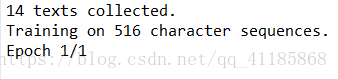+关注继续查看

RNN 循环神经网络系列 2：文本分类

预处理步骤

1. 清洗句子并切分成一个个 token；
2. 将句子转换为数值 token；
3. 保存每个句子的序列长。Screen Shot 2016-10-05 at 7.32.36 PM.png

模型

class model(object):

def __init__(self, FLAGS):

# 占位符
self.inputs_X = tf.placeholder(tf.int32,
shape=[None, None], name='inputs_X')
self.targets_y = tf.placeholder(tf.float32,
shape=[None, None], name='targets_y')
self.dropout = tf.placeholder(tf.float32)

# RNN 单元
stacked_cell = rnn_cell(FLAGS, self.dropout)

# RNN 输入
with tf.variable_scope('rnn_inputs'):
W_input = tf.get_variable("W_input",
[FLAGS.en_vocab_size, FLAGS.num_hidden_units])

inputs = rnn_inputs(FLAGS, self.inputs_X)
#initial_state = stacked_cell.zero_state(FLAGS.batch_size, tf.float32)

# RNN 输出
seq_lens = length(self.inputs_X)
all_outputs, state = tf.nn.dynamic_rnn(cell=stacked_cell, inputs=inputs,
sequence_length=seq_lens, dtype=tf.float32)

# 由于使用了 seq_len，state 自动包含了上一次的对应输出
# 因为 state 是一个带有张量的元组
outputs = state

# 处理 RNN 输出
with tf.variable_scope('rnn_softmax'):
W_softmax = tf.get_variable("W_softmax",
[FLAGS.num_hidden_units, FLAGS.num_classes])
b_softmax = tf.get_variable("b_softmax", [FLAGS.num_classes])

# Logits
logits = rnn_softmax(FLAGS, outputs)
probabilities = tf.nn.softmax(logits)
self.accuracy = tf.equal(tf.argmax(
self.targets_y,1), tf.argmax(logits,1))

# 损失函数
self.loss = tf.reduce_mean(
tf.nn.sigmoid_cross_entropy_with_logits(logits, self.targets_y))

# 优化
self.lr = tf.Variable(0.0, trainable=False)
trainable_vars = tf.trainable_variables()
# 使用梯度截断来避免梯度消失和梯度爆炸

# 下面是用于采样的值
# (在每个单词后生成情绪)

# 取所有输出作为第一个输入序列
# (由于采样，只需一个输入序列)
sampling_outputs = all_outputs

# Logits
sampling_logits = rnn_softmax(FLAGS, sampling_outputs)
self.sampling_probabilities = tf.nn.softmax(sampling_logits)

# 保存模型的组件
self.global_step = tf.Variable(0, trainable=False)
self.saver = tf.train.Saver(tf.all_variables())

def step(self, sess, batch_X, batch_y=None, dropout=0.0,
forward_only=True, sampling=False):

input_feed = {self.inputs_X: batch_X,
self.targets_y: batch_y,
self.dropout: dropout}

if forward_only:
if not sampling:
output_feed = [self.loss,
self.accuracy]
elif sampling:
input_feed = {self.inputs_X: batch_X,
self.dropout: dropout}
output_feed = [self.sampling_probabilities]
else: # 训练
output_feed = [self.train_optimizer,
self.loss,
self.accuracy]

outputs = sess.run(output_feed, input_feed)

if forward_only:
if not sampling:
return outputs, outputs
elif sampling:
return outputs
else: # 训练
return outputs, outputs, outputs

def length(data):
relevant = tf.sign(tf.abs(data))
length = tf.reduce_sum(relevant, reduction_indices=1)
length = tf.cast(length, tf.int32)
return length

推断Screen Shot 2016-10-05 at 8.34.51 PM.png

损失屏蔽（这里不需要）

# 向量化 logits 和目标
targets = tf.reshape(targets, [-1]) # 将张量 targets 转为向量
losses = tf.nn.sparse_softmax_cross_entropy_with_logits(logits, targets)
mask = tf.sign.(tf.to_float(targets)) # targets 为 0 则输出为 0, target < 0 则输出为 -1, 否则 为 1

代码

GitHub 仓库 （正在更新，敬请期待！）

张量形状变化的参考10095 02963 01861 010886 013893 0py之textgenrnn：Python利用textgenrnn库实现训练文本生成网络
py之textgenrnn：Python利用textgenrnn库实现训练文本生成网络
17 02940 011893 07365 02161 0

20710

438

《2021云上架构与运维峰会演讲合集》

《零基础CSS入门教程》

《零基础HTML入门教程》